# Thermochemical Hydrocarbon Splitting

## General Hydrocarbons

The general hydro-carbon $$C_nH_m$$ source for hydrogen results from the general steam-reformation reaction $$C_nH_m + n H_2O \rightarrow n CO + (n + m/2) H_2$$ to deliver pure diatomic hydrogen $$H_2$$.

## Methane

Natural Gas is mostly Methane $$CH_4$$ which is the hydrogen source material for the steam-reformation reaction $$CH_4 + H_2O \rightarrow CO + 3 H_2$$ to deliver pure diatomic hydrogen $$H_2$$.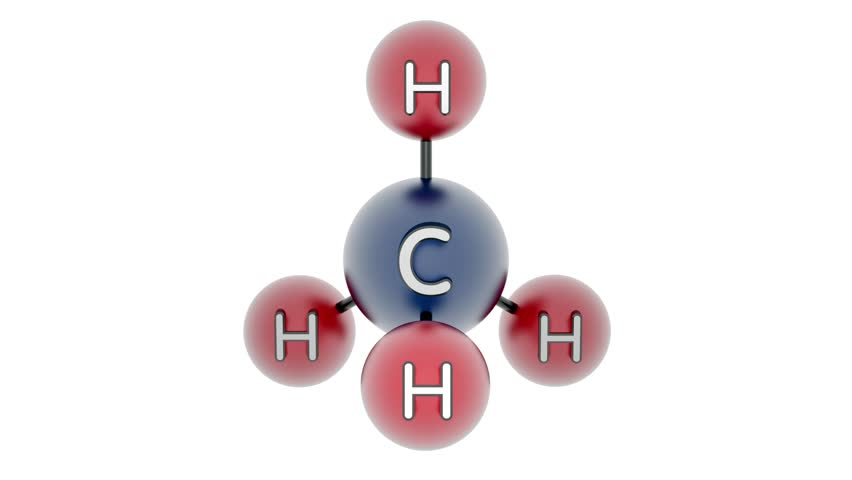++ 3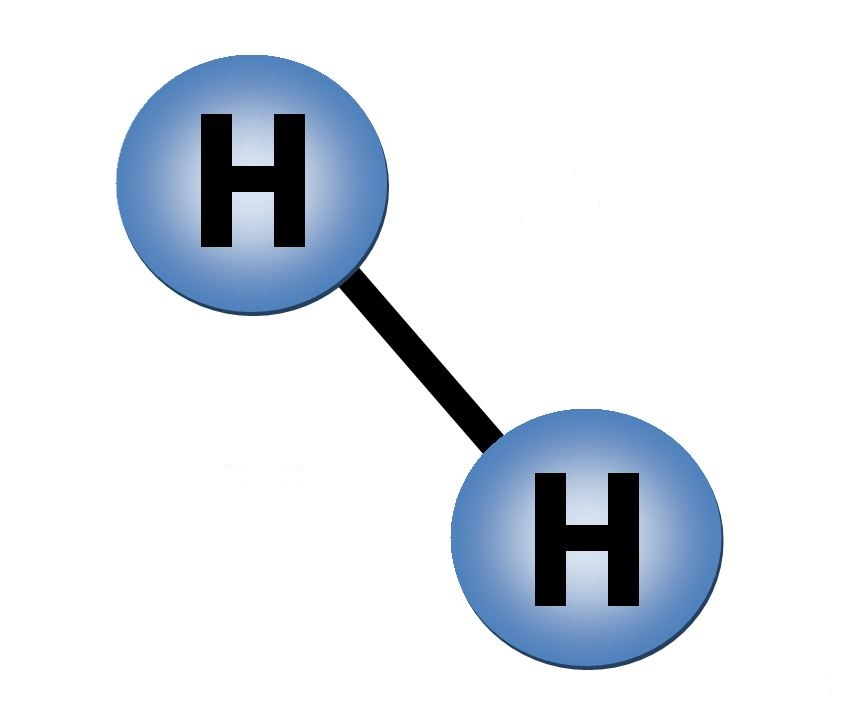## Methanol

For the case of Wood alcohol or Methanol $$CH_3OH$$ as hydrogen source material the steam-reformation reaction $$CH_3OH + H_2O \rightarrow CO + 3 H_2$$ delivers the pure diatomic hydrogen $$H_2$$.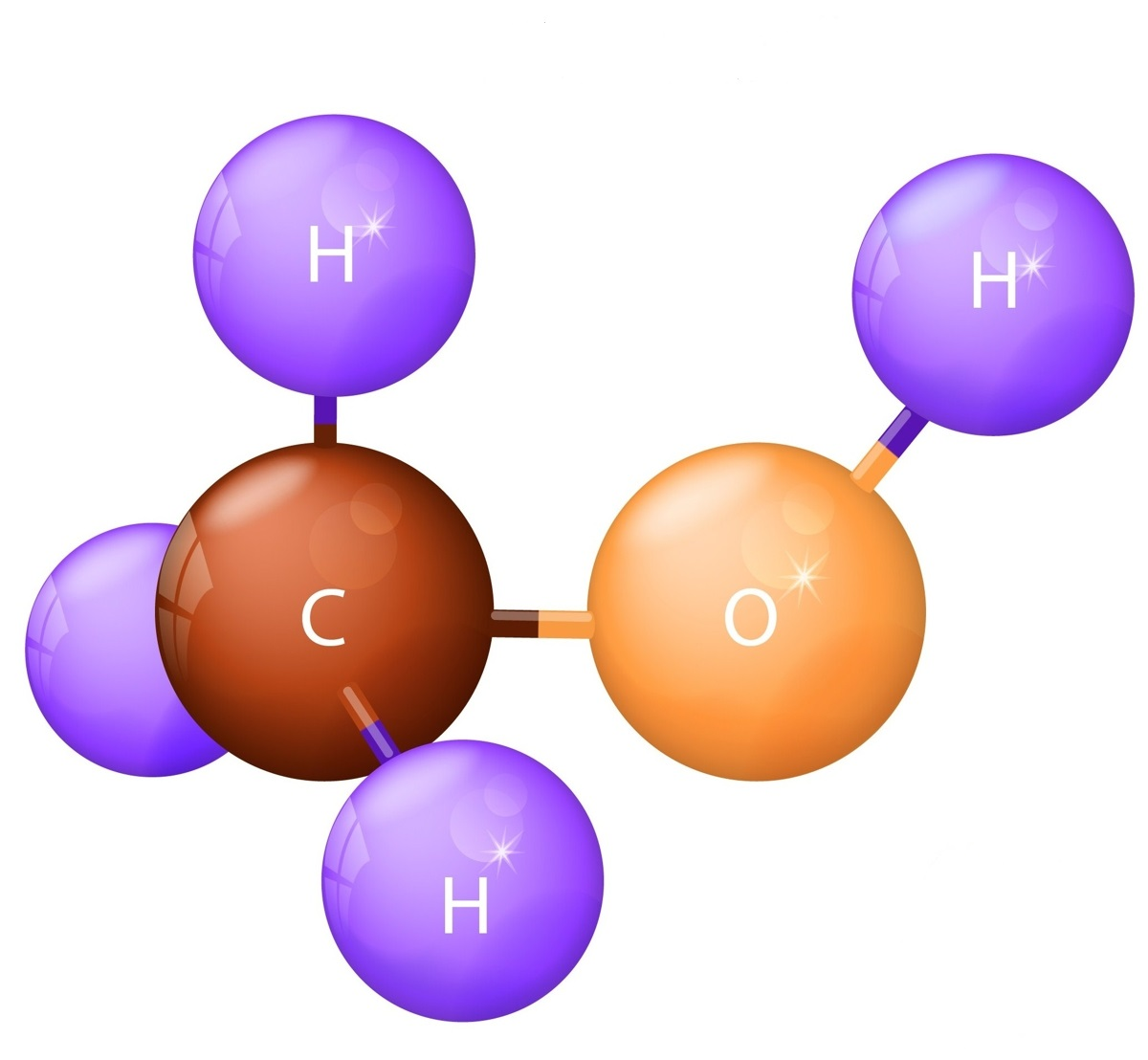++ 3## Propane

For the case of Propane $$C_3H_8$$ as hydrogen source material the steam-reformation reaction $$C_3H_8 + 3 H_2O \rightarrow 3 CO + 7 H_2$$ delivers the pure diatomic hydrogen $$H_2$$.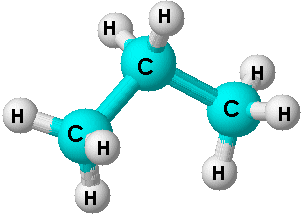+ 3→ 3+ 7## Water-Gas Shift Reaction

The water-gas shift reaction $$CO + H_2O \rightarrow CO_2 + H_2$$ then further converts the $$CO$$ into $$CO_2$$ by water splitting to generate even more hydrogen.+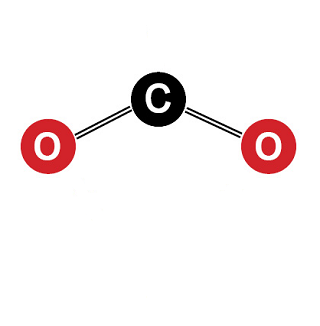+Website created by Off Grid Home Supply IT Dept